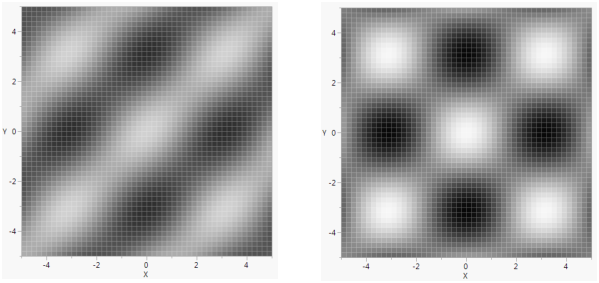Publication date: 08/13/2020

## Plot Functions

A Y Function() function is used to draw smooth functions. The first argument is the expression to be plotted. The second argument is the name of the X variable in the expression.

`win = New Window( "Sine Function",`
`	Graph Box(`
`		Frame Size( 200, 100 ),`
`		X Scale( -10, 10 ),`
`		Y Scale( -1, 1 ),`
`		X Name( "x" ),`
`		Y Name( "Sine(x)" ),`
`		Y Function( Sine( x ), x ) ) );`

Figure 12.32 Sine Wave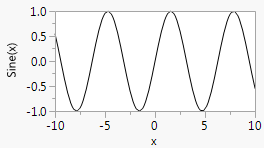You can use For to overlap several sine waves:

`win = New Window( "Overlapping Sine Waves",`
`	Graph Box(`
`		Frame Size( 200, 100 ),`
`		X Scale( -10, 10 ),`
`		Y Scale( -1, 1 ),`
`		For( i = 1, i <= 4, i += .1,`
`			Y Function( Sine( x / i ), x )`
`		)`
`	)`
`);`

Figure 12.33 Overlapping Sine Waves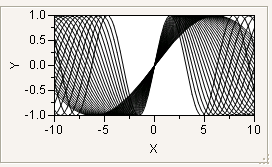Similarly, X Function() is for drawing a graph where the symbol is varied on the Y variable.

`win = New Window( "Overlapping Sine Waves",`
`	Graph Box(`
`		Frame Size( 100, 200 ),`
`		X Scale( -1, 1 ),`
`		Y Scale( -10, 10 ),`
`		For( i = 1, i <= 4, i += .2,`
`			X Function( Sine( y / i ), y )`
`		)`
`	)`
`);`

Figure 12.34 Overlapping Sine Waves along the X-Axis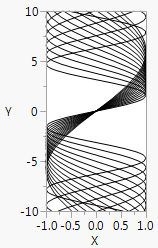An XY Function() draws a smooth curve using a pair of formulas (parametric equations) that depend on a third variable. The third variable’s value is incremented from a minimum value to a maximum value to generate the X-Y pairs.

`win = New Window( "Spiral",`
`	Graph Box(`
`		Pen Color( "red" ); // red line color`
`		xCenter = 50; // location of the center of the curve on the x axis`
`		yCenter = 50; // location of the center of the curve on the y axis`
`		minAngle = 0;`
`		maxAngle = Pi() * 2 * 20;`
`		XY Function(`
`			xCenter + ((ta / 3) * Cos( ta )),`
`			yCenter + ((ta / 3) * Sin( ta )),`
`			ta,`
`			Min( minAngle ),`
`			Max( maxAngle ),`
`			Inc( Pi() / 100 )`
`		);`
`	)`
`);`

In this example, Sin() and Cos() use ta as an argument (rotates) and as a factor (expands). (Sin() and Cos() use radians, not degrees.)

Figure 12.35 Spiral Parametric Plot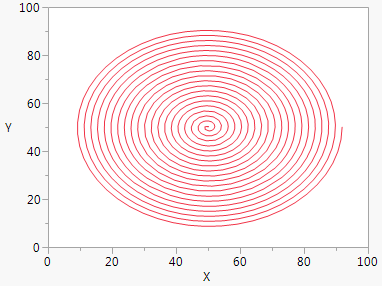Contour Function() is an analogous way to represent a three-dimensional function in a two-dimensional space. The final argument specifies the value(s) for the contour line(s). The argument can be a value, an indexed range of values using ::, or a matrix of values.

`win = New Window( "Bird's eye view of the egg carton function",`
`	Graph Box(`
`		Frame Size( 300, 300 ),`
`		X Scale( -10, 10 ),`
`		Y Scale( -10, 10 ),`
`		Pen Color( "black" );`
`		Pen Size( 2 );`
`		Contour Function( Sine( y ) + Cosine( x ), x, y, (0 :: 20) / 5 );`
`		Pen Color( "red" );`
`		Pen Size( 1 );`
`		Contour Function( Sine( y ) + Cosine( x ), x, y, (-20 :: 0) / 5 );`
`	)`
`);`

Figure 12.36 Egg Carton Function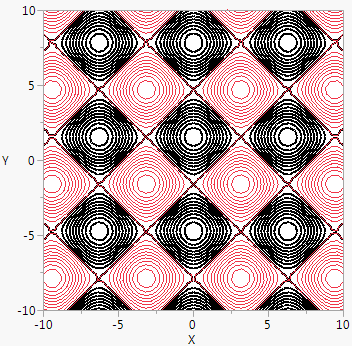Normal Contour() draws normal probability contours for k populations and two variables. The first argument is a scalar probability or a matrix of probability values for the contours. Subsequent arguments are matrices to specify means, standard deviations, and correlations. The mean and standard deviation matrices have dimension k × 2. The correlation matrix should be k × 1, where the first row pertains to the first contour, the second row to the second contour, and so on. The first column is for x and the second column for y. Consider the following example:

`Normal Contour(`
`	[ prob1,`
`	  prob2,`
`	  prob3, ...],`
`	[ xmean1 ymean1,`
`	  xmean2 ymean2,`
`	  xmean3 ymean3, ...],`
`	[ xsd1 ysd1,`
`	  xsd2 ysd2,`
`	  xsd3 ysd3, ...],`
`	[ xycorr1,`
`	  xycorr2,`
`    xycorr3, ...]);`

The following script draws contours at probabilities 0.1, 0.5, 0.7, and 0.99 for two populations and two variables. The first population has x mean 0 and y mean 1, with standard deviation 0.3 along the x axis and 0.6 along the y-axis, and with correlation 0.5. The second has x mean 4 and y mean 6, with standard deviation 0.8 along the x axis and 0.4 along the y-axis, and with correlation 0.9.

`win = New Window( "Normal Contours",`
`	Graph Box(`
`		X Scale( -5, 10 ),`
`		Y Scale( -5, 10 ),`
`		Normal Contour( [.1, .5, .7, .99], [0 1, 4 6], [.3 .6, .8 .4], [.5, .9] )`
`	)`
`);`

Figure 12.37 Normal Contour Function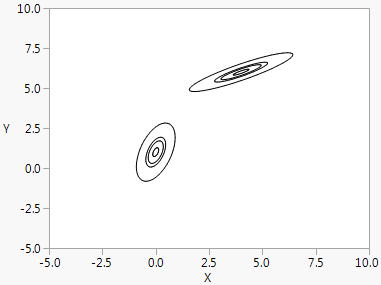Normal Contour() is a general way to accomplish effects such as Bivariate’s density ellipses. The Bivariate script in the Football.jmp sample data creates an example.

Gradient Function() fills a set of rectangles on a grid according to a color determined by the expression value as it crosses a range corresponding to a range of colors.

`Gradient Function( expression, xname, yname, [zlow, zhigh], ZColor( [colorLow, colorHigh] ), <XGrid( min, max, incr )>, <YGrid( min, max, incr )>, <Transparency( t )>;`

Here are explanations of the elements in the Gradient Function() syntax:

`GradientFunction(`
`	/* the expression to be contoured, which is a function in terms of`
`	the two variables that follow expression*/`
`	Expression,`
` `
`	// the two variable names used in the expression`
`	xname, yname,`
` `
`	// the low and high expression values the gradient is scaled between`
`	[zlow, zhigh],`
` `
`	// the colors that correspond to the low value and the high value`
`	ZColor([colorLow, colorHigh])`
` `
`// optional specification for the grid of values`
`<XGrid(min, max, incr),>`
`<YGrid(min, max, incr)> );`
` `
`/* the value 0 means clear. The value 1 means completely opaque and`
`is the usual drawing mode. */`
`<Transparency>`

The ZColor() values must be numeric codes rather than names. You can use the color menu indices (such as 0=black, 1=grey, 2=white, 3=red, 4=green, and 5=blue) found in Specify Colors.

The following example uses Gradient Function() to create an animated graph.

`phase = 0.7;`
`win = New Window( "Gradient Function",`
`	a = Graph(`
`		Frame Size( 400, 400 ),`
`		X Scale( -5, 5 ),`
`		Y Scale( -5, 5 ),`
`		Gradient Function(`
`			phase * Sine( x ) * Sine( y ) + (1 - phase) * Cosine( x ) * Cosine( y ),`
`			x,`
`			y,`
`			[-1 1],`
`			zcolor( [0, 2] )`
`		)`
`	)`
`);`
`b = a[FrameBox( 1 )];`
`For( i = 1, i <= 5, i++,`
`	For( phase = 0, phase < 1, phase += 0.05,`
`		b << Reshow;`
`		Wait( 0.01 );`
`	);`
`	For( phase = 1, phase > 0, phase -= 0.05,`
`		b << Reshow;`
`		Wait( 0.01 );`
`	);`
`);`